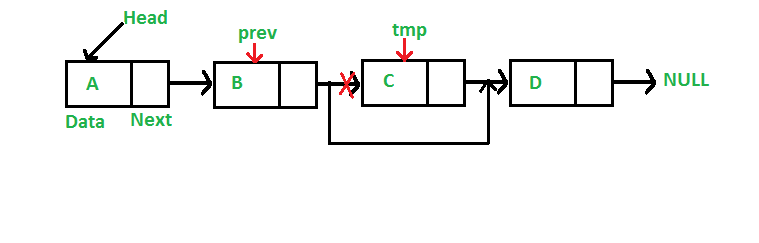# 7.10: Linked List Node Delete

$$\newcommand{\vecs}{\overset { \rightharpoonup} {\mathbf{#1}} }$$ $$\newcommand{\vecd}{\overset{-\!-\!\rightharpoonup}{\vphantom{a}\smash {#1}}}$$$$\newcommand{\id}{\mathrm{id}}$$ $$\newcommand{\Span}{\mathrm{span}}$$ $$\newcommand{\kernel}{\mathrm{null}\,}$$ $$\newcommand{\range}{\mathrm{range}\,}$$ $$\newcommand{\RealPart}{\mathrm{Re}}$$ $$\newcommand{\ImaginaryPart}{\mathrm{Im}}$$ $$\newcommand{\Argument}{\mathrm{Arg}}$$ $$\newcommand{\norm}{\| #1 \|}$$ $$\newcommand{\inner}{\langle #1, #2 \rangle}$$ $$\newcommand{\Span}{\mathrm{span}}$$ $$\newcommand{\id}{\mathrm{id}}$$ $$\newcommand{\Span}{\mathrm{span}}$$ $$\newcommand{\kernel}{\mathrm{null}\,}$$ $$\newcommand{\range}{\mathrm{range}\,}$$ $$\newcommand{\RealPart}{\mathrm{Re}}$$ $$\newcommand{\ImaginaryPart}{\mathrm{Im}}$$ $$\newcommand{\Argument}{\mathrm{Arg}}$$ $$\newcommand{\norm}{\| #1 \|}$$ $$\newcommand{\inner}{\langle #1, #2 \rangle}$$ $$\newcommand{\Span}{\mathrm{span}}$$$$\newcommand{\AA}{\unicode[.8,0]{x212B}}$$

## Delete Node

We have looked at creating a linked list, and we have worked through inserting a new node into a linked list. Now we will work through deleting a node from the list.

To delete a node from linked list, we need to do following steps.
1) Find previous node of the node to be deleted.
2) Change the next of previous node.
3) Free memory for the node to be deleted.In this instance we remove the node where C is the Data. This simply means we reset the B node Next value to point the the D node. Remember: the Next value is a memory pointer that points the the place in memory where the various structures exist. Then we release the memory, using the free() function,  location where the C node was.

The following code uses a struct instead of a Class, this allows you to see a slightly different way to implement linked lists. This is a fully implemented linked list using structures. Pay attention to the printList() function. Again, this is an example of walking across the list. Taking the head, which is the argument passed into this function, and seeing if it points to NULL. As long as it points to a valid address we print the Data member, and reset the node pointer to the current Next, then go back to the while statement.

// A complete working C program to demonstrate deletion in singly
#include <iostream>
using namespace std;

struct Node
{
char data;
struct Node *next;
};

/* Given a reference (pointer to pointer) to the head of a list
and a char, inserts a new node on the front of the list. */
void push(struct Node** head_ref, char new_data)
{
struct Node* new_node = new Node;
new_node->data = new_data;
}

/* Given a reference (pointer to pointer) to the head of a list
and a key, deletes the first occurrence of key in linked list */
void deleteNode(struct Node **head_ref, char key)
{
struct Node* temp = *head_ref, *prev;
// If head node itself holds the key to be deleted
if (temp != NULL && temp->data == key)
{
delete(temp);             // free old head memory
return;
}

// Search for the key to be deleted, keep track of the
// previous node as we need to change 'prev->next'
while (temp != NULL && temp->data != key)
{
prev = temp;
temp = temp->next;
}

// If key was not present in linked list
if (temp == NULL)
return;
prev->next = temp->next;
delete(temp); // Free memory
}

// This function prints contents of linked list starting from
// the given node
void printList(struct Node *node)
{
while (node != NULL)
{
cout << node->data;
node = node->next;
}
cout << endl;
}

/* Main to test above functions*/
int main()
{

cout << "Created Linked List: " << endl;
cout << "Linked List after Deletion of 'C': " << endl;
return 0;
}

Output:

Created Linked List:
DCBA
Linked List after Deletion of 'C':
DCA